# 8.2 Rule 2: Trace placement parallel and close to metallic enclosure walls reduces EMI, trace placement orthogonal and close to enclosure walls increases EMI

Figure 8.2(b) shows a radiated power reduction of 20dB over the whole frequency range from 100MHz to 4GHz when the trace placement is changed from that of Trace A to that of Trace B, as depicted in Figure 8.2(a). It is recommended to place critical traces parallel and close to the metallic enclosure walls. This must already be considered, when placing the driver and receiver ICs on the PCB.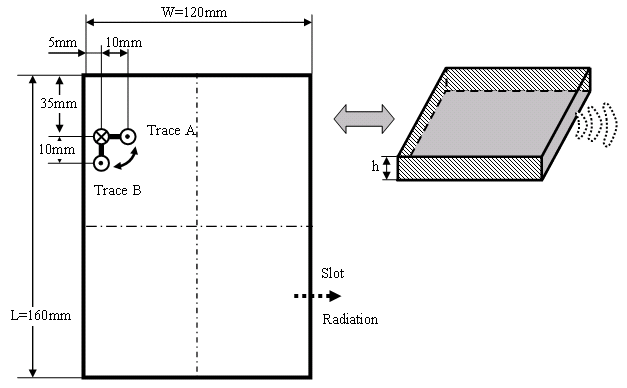(a) Placement of Trace A and Trace B.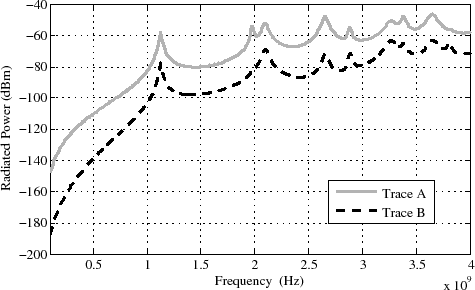(b) Overall radiated power from the enclosure slot.
Figure 8.2: The trace width is 0.2mm, the trace height above the ground plane is 0.65mm. A 10mV voltage source with an impedance equal to the characteristic impedance of the trace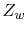drives the trace which is terminated with a 10pF capacitance.

Trace B in Figure 8.2(a) is closer to the back wall than Trace A. Therefore, it cannot be determined which part of the emission reduction in Figure 8.2(b) is obtained from the closer placement and which part from the parallel placement of Trace B to the enclosure wall. Therefore, Figure 8.3 depicts the comparison with a Trace B parallel to the enclosure wall at the maximum distance of Trace A from that wall. The result of the comparison in Figure 8.3(b) shows that 10dB of the 20dB in Figure 8.2(b) reduction can be assigned to the parallel placement and 10dB to the closer placement.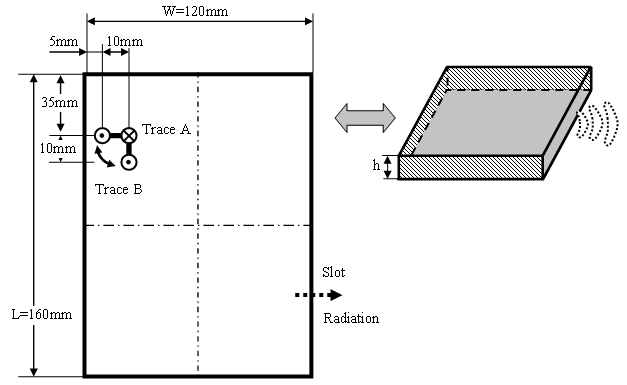(a) Placement of Trace A and Trace B.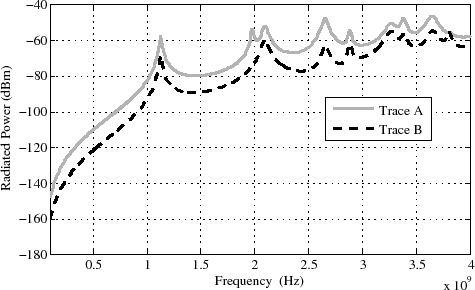(b) Overall radiated power from the enclosure slot.
Figure 8.3: The trace width is 0.2mm, the trace height above the ground plane is 0.65mm. A 10mV voltage source with an impedance equal to the characteristic impedance of the tracedrives the trace which is terminated with a 10pF capacitance.

The same emission reduction of 20dB is also observed in Figure 8.4, when the Trace B is arranged closer and parallel to a side wall of the enclosure.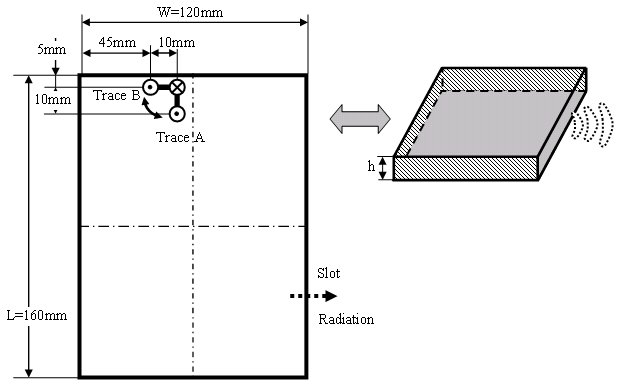(a) Placement of Trace A and Trace B.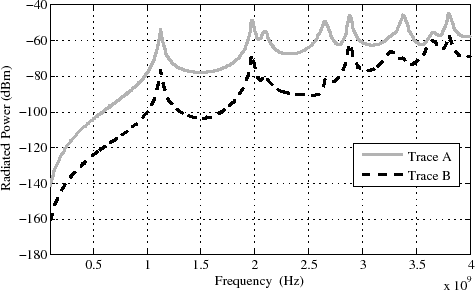(b) Overall radiated power from the enclosure slot.
Figure 8.4: The trace width is 0.2mm, the trace height above the ground plane is 0.65mm. A 10mV voltage source with an impedance equal to the characteristic impedance of the tracedrives the trace which is terminated with a 10pF capacitance.

A comparison with a Trace B parallel to the enclosure wall at the maximum distance of Trace A from that wall in Figure 8.5 shows a similar result as for the back wall. Nearly 10dB emission reduction is achieved with a closer placement of the trace to the enclosure wall and 10dB reduction results from the orientation of the trace parallel to the wall.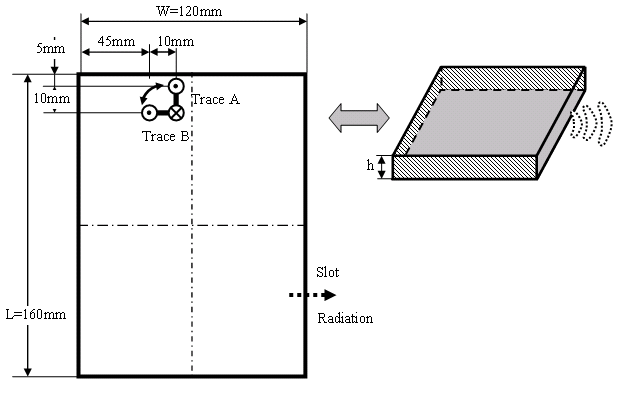(a) Placement of Trace A and Trace B.(b) Overall radiated power from the enclosure slot.
Figure 8.5: The trace width is 0.2mm, the trace height above the ground plane is 0.65mm. A 10mV voltage source with an impedance equal to the characteristic impedance of the tracedrives the trace which is terminated with a 10pF capacitance.

The design rule is generalized to fairly arbitrary shaped, and even electrically large metallic enclosures as follows. The field inside a metallic enclosure can generally be described by an eigenfunction superposition. Since the eigenfunctions can be obtained from a homogenous solution of the Maxwell equations, the following investigation of the field close to a metallic enclosure wall is carried out from the homogenous Maxwell equations. A smooth metallic enclosure surface can be approximated locally by the tangential plane. The fields close to a metallic plane are expressed in a coordinate system with one coordinate direction normal (index ^) to the plane and two coordinate directions parallel (index ||) to the plane. The electric field, the magnetic field, and the vector operator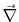are expressed within this coordinate system. With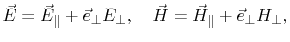and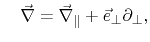(8.1)

the Maxwell curl equations for the electric field become(8.2)

and the Maxwell curl equations for the magnetic field become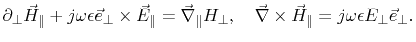(8.3)

From the Maxwell divergence equations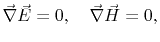(8.4)

follows(8.5)

With consideration of the PEC boundary at the metallic plane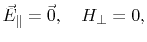(8.6)

and (8.3) follows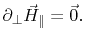(8.7)

Considering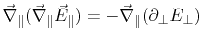(8.8)

from (8.5), the normal derivative of (8.2) becomes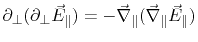(8.9)

Directly on the metallic plane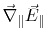vanishes, because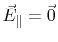constant along the plane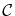, and (8.9) becomes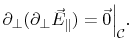(8.10)

Thus, the normal derivative of the field component parallel to the metallic plane has a maximum at this plane, which is expressed by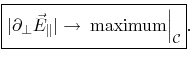(8.11)

The tangential electric field vanishes at the metallic plane and the derivative of the field becomes a maximum. Consequently, a differential coupling to that field must reach a maximum, when a trace is oriented orthogonal to the wall, because the coupling of a differential source depends on the field derivative. Close to the walls, emission reduction reaches a maximum according to the vanishing tangential electric field. For the enclosure depicted in Figure 7.1, this also follows from the interpretation of (7.17).

C. Poschalko: The Simulation of Emission from Printed Circuit Boards under a Metallic Cover